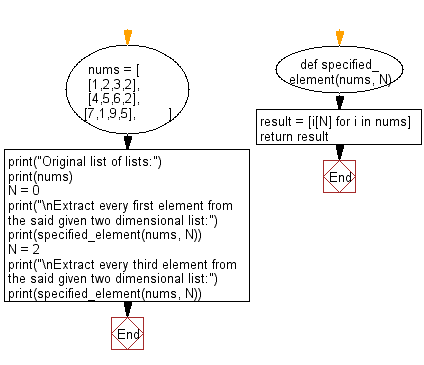﻿ Python: Extract every first or specified element from a given two-dimensional list - w3resource# Python: Extract every first or specified element from a given two-dimensional list

## Python List: Exercise - 144 with Solution

Write a Python program to extract every first or specified element from a given two-dimensional list.

Sample Solution:

Python Code:

``````def specified_element(nums, N):
result = [i[N] for i in nums]
return result

nums = [
[1,2,3,2],
[4,5,6,2],
[7,1,9,5],
]

print("Original list of lists:")
print(nums)
N = 0
print("\nExtract every first element from the said given two dimensional list:")
print(specified_element(nums, N))
N = 2
print("\nExtract every third element from the said given two dimensional list:")
print(specified_element(nums, N))
```
```

Sample Output:

```Original list of lists:
[[1, 2, 3, 2], [4, 5, 6, 2], [7, 1, 9, 5]]

Extract every first element from the said given two dimensional list:
[1, 4, 7]

Extract every third element from the said given two dimensional list:
[3, 6, 9]
```

Flowchart:## Visualize Python code execution:

The following tool visualize what the computer is doing step-by-step as it executes the said program:

Python Code Editor:

Have another way to solve this solution? Contribute your code (and comments) through Disqus.

What is the difficulty level of this exercise?

Test your Python skills with w3resource's quiz

﻿

## Python: Tips of the Day

Floor Division:

When we speak of division we normally mean (/) float division operator, this will give a precise result in float format with decimals.

For a rounded integer result there is (//) floor division operator in Python. Floor division will only give integer results that are round numbers.

```print(1000 // 300)
print(1000 / 300)```

Output:

```3
3.3333333333333335```